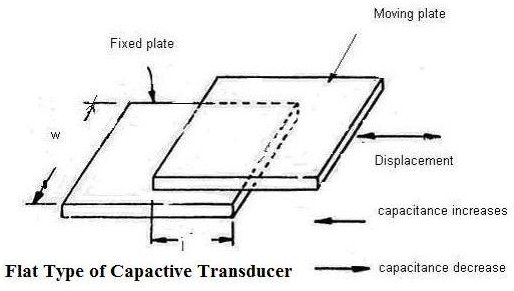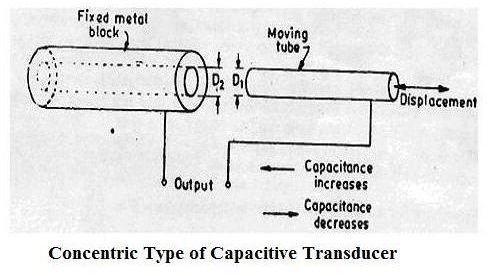# How Capacitive Transducers Works? Capacitive Sensors. Variable Capacitance Transducers

## What is Capacitve Transducer?

The capacitive transducer or sensor is nothing but the capacitor with variable capacitance. The capacitive transducer comprises of two parallel metal plates that are separated by the material such as air, which is called as the dielectric material. In the typical capacitor the distance between the two plates is fixed, but in variable capacitance transducers the distance between the two plates is variable.

In the instruments using capacitance transducers the value of the capacitance changes due to change in the value of the input quantity that is to be measured. This change in capacitance can be measured easily and it is calibrated against the input quantity, thus the value if the input quantity can be measured directly.

## Capactive Transducer or Capacitive Sensor or Variable Capacitance Transducer## Capacitance of the Capacitive Transducers

The capacitance C between the two plates of capacitive transducers is given by:

C = εo x εr x A/ d

Where C is the capacitance of the capacitor or the variable capacitance transducer

εo is the absolute permittivity

εr is the relative permittivity

The product of εo & εr is also called as the dielectric constant of the capacitive transducer.

A is the area of the plates

D is the distance between the plates

It is clear from the above formula that capacitance of the capacitive transducer depends on the area of the plates and the distance between the plates. The capacitance of the capacitive transducer also changes with the dielectric constant of the dielectric material used in it.

Thus the capacitance of the variable capacitance transducer can change with the change of the dielectric material, change in the area of the plates and the distance between the plates. Depending on the parameter that changes for the capacitive transducers, they are of three types as mentioned below.

## 1) Changing Dielectric Constant type of Capacitive Transducers

In these capacitive transducer the dielectric material between the two plates changes, due to which the capacitance of the transducer also changes. When the input quantity to be measured changes the value of the dielectric constant also changes so the capacitance of the instrument changes. This capacitance, calibrated against the input quantity, directly gives the value of the quantity to be measured. This principle is used for measurement of level in the hydrogen container, where the change in level of hydrogen between the two plates results in change of the dielectric constant of the capacitance transducer. Apart from level, this principle can also be used for measurement of humidity and moisture content of the air.

## 2) Changing Area of the Plates of Capacitive Transducers

The capacitance of the variable capacitance transducer also changes with the area of the two plates. This principle is used in the torquemeter, used for measurement of the torque on the shaft. This comprises of the sleeve that has teeth cut axially and the matching shaft that has similar teeth at its periphery.

## 3) Changing Distance between the Plates of Capacitive Transducers

In these capacitive transducers the distance between the plates is variable, while the area of the plates and the dielectric constant remain constant. This is the most commonly used type of variable capacitance transducer. For measurement of the displacement of the object, one plate of the capacitance transducer is kept fixed, while the other is connected to the object. When the object moves, the plate of the capacitance transducer also moves, this results in change in distance between the two plates and the change in the capacitance. The changed capacitance is measured easily and it calibrated against the input quantity, which is displacement. This principle can also be used to measure pressure, velocity, acceleration etc.